A point is used for marking position; it has neither length, breadth, nor thickness.

## Lines

A line has length only; it is produced by the motion of a point.

A straight line or right line is one that has the same direction throughout. It is the shortest distance between two points.

A curved line is one that is constantly changing in direction. It is sometimes called a curve.

A broken line is one made up of several straight lines.

Parallel lines are lines which lie in the same plane and are equally distant from each other at all points.

A horizontal line is one having the direction of a line drawn upon the surface of water that is at rest. It is a line parallel to the horizon.

A vertical line is one that lies in the direction of a thread suspended from its upper end and having a weight at the lower end. It is a line that is perpendicular to a horizontal plane.

An oblique line is one that is neither vertical nor horizontal.

In Mechanical Drawing, lines drawn along the edge of the T-square, when the head of the T-square is resting against the left-hand edge of the board, are called horizontal lines. Those drawn at right angles or perpendicular to the edge of the T-square are called vertical lines.

If two lines cut each other, they are called intersecting lines, and the point at which they cross is called the point of intersection.

Copyright, 1911, by American School of Correspondence.

## Angles

An angle is the measure of the difference in direction of two lines. The lines are called sides, and the point of meeting, the vertex. The size of an angle is independent of the length of the lines.

If one straight line meets another (extended if necessary), Fig. 40, so that the two angles thus formed are equal, the lines are said to be perpendicular to each other and the angles formed are called right angles.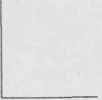Fig. 40. Right Angle.Fig. 41. Acute Angle.Fig. 42. Obtuse Angle.

An acute angle is less than a right angle, Fig. 41.

An obtuse angle is greater than a right angle, Fig. 42.

## Surfaces

A surface is produced by the motion of a line; it has two dimensions - length and breadth.

A plane figure is a plane bounded on all sides by lines; the space included within these lines (if they are straight lines) is called a polygon or a rectilinear figure.

## Polygons

A polygon is a plane figure bounded by straight lines. The boundary lines are called the sides and the sum of the sides is called the perimeter.Fig. 13. Pentagon.Fig. 44. Hexagon.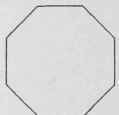Fig. 45. Octagon.

Polygons are classified according to the number of sides.

A triangle is a polygon of three sides.

A quadrilateral is a polygon of four sides.

A pentagon is a polygon of five sides, Fig. 43.

A hexagon is a polygon of six sides, Fig. 44.

A heptagon is a polygon of seven sides.

An octagon is a polygon of eight sides, Fig. 45.

A decagon is a polygon of ten sides.

A dodecagon is a polygon of twelve sides.

An equilateral polygon is one all of whose sides are equal.

An equiangular polygon is one all of whose angles are equal.

A regular polygon is one all of whose angles and all of whose sides are equal.

Triangles. A triangle is a polygon enclosed by three straight lines called sides. The angles of a triangle are the angles formed by the sides.

A right-angled triangle, often called a right triangle, Fig. 46, is one that has a right angle. The longest side (the one opposite the right angle) is called the hypotenuse, and the other sides are sometimes called legs.Fig. 4G. Right-Angled Triangle-'.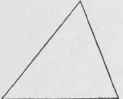Fig. 47. Acute-Angled Triangle.Fig. 48. Obtuse-Angled Triangle.

An acute-angled triangle is one that has all of its angles acute, Fig. 47.

An obtuse-angled triangle is one that has an obtuse angle, Fig. 48.Fig. 43. Equilateral Triangle.Fig. 50. Isosceles Triangle.Fig. 51. Scalene Triangle.

An equilateral triangle is one having all of its sides equal, Fig. 49. An equiangular triangle is one having all of its angles equal. An isosceles triangle, Fig. 50, is one, two of whose sides are equal. A scalene triangle, Fig. 51, is one, no two of whose sides are equal.

The base of a triangle is the lowest side; it is the side upon which the triangle is supposed to stand. Any side may, however, be taken as the base. In an isosceles triangle, the side which is not one of the equal sides is usually considered as the base.

The altitude of a triangle is the perpendicular drawn from the vertex to the base.

Quadrilaterals. A quadrilateral is a polygon bounded by four straight lines, as Fig. 52.

The diagonal of a quadrilateral is a straight line joining two opposite vertices.

Trapezium. A trapezium is a quadrilateral, no two of whose sides are parallel.

Trapezoid. A trapezoid is a quadrilateral having two sides parallel, Fig. 53. ' The parallel sides are called the bases and the perpendicular distance between the bases is called the altitude.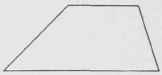Fig. 53. Trapezoid.Fig. 54. Parallelogram.

Parallelogram. A parallelogram is a quadrilateral whose opposite sides are parallel, Fig. 54.

There are four kinds of parallelograms: rectangle, square, rhombus, and rhomboid.

The rectangle, Fig. 55, is a parallelogram whose angles are right angles.Fig. 55. Rectangle.Fig. 56. Square.Fig. 57. Rhombus.

The square, Fig. 56, is a parallelogram all of whose sides are equal and whose angles are right angles.

The rhombus, Fig. 57, is a parallelogram whose sides are equal but whose angles are not right angles.

The rhomboid is a parallelogram whose adjacent sides are unequal, and whose angles are not right angles.

## Circles

A circle is a plane figure bounded by a curved line called the circumference, every point of which is equally distant from a point within called the center, Fig. 58.

A diameter of a circle is a straight line drawn through the center, terminating; at both ends in the circumference. Fig. 59.Fig. 58. Circle.Fig. 59. Diameter and Tangent.A radius of a circle is a straight line joining the center with the circumference. All radii of the same circle are equal and their length is always one-half that of the diameter.

An arc is any part of the circumference of a circle. An arc equal to one-half the circumference is called a semi-circumference, and an arc equal to one-quarter of the circumference is called a quadrant, Fig. 60. A quadrant may mean the arc or angle.

A chord, Fig. 61, is a straight line which joins the extremities of an arc but does not pass through the center of the circle.

A secant is a straight line which intersects the circumference in two points, Fig. 61.

A segment of a circle, Fig. 62, is the area included between an arc and a chord.

A sector is the area included between an arc and two radii drawn to the extremities of the arc, Fig. 62.Fig. 61. Chord and Secant.Fig. 62 Segment and Sector.Fig. 63. Concentric Circles.

A tangent is a straight line which touches the circumference at only one point, called the point of tangency or contact, Fig. 59.

Concentric circles are circles having the same center, Fig. 63.

An inscribed angle is an angle whose vertex lies in the circum-ference and whose sides are chords. It is measured by one-half the intercepted arc, Fig. 64.Fig. 64. Inscribed Angle.Fig. 65. Central Angle.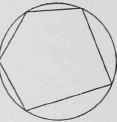Fig. 66. Inscribed Polygon.

A central angle is an angle whose vertex is at the center of the circle and whose sides are radii, Fig. 65.

An inscribed polygon is one whose vertices lie in the circumference and whose sides are chords, Fig. 66.

## Measurement Of Angles

To measure an angle, take any convenient radius and describe an arc with the center at the vertex of the angle. The portion of the arc included between the sides of the angle is the measure of the angle. If the arc has a constant radius, the greater the divergence of the sides, the longer will be the arc. If there are several arcs drawn with the same center, the intercepted arcs will have different lengths but they will all be the same fraction of the entire circumference.

In order that the size of an angle or arc may be stated without saying that it is a certain fraction of a circumference, the circumference is divided into 360 equal parts called degrees, Fig. 67. Thus, it may be said that a certain angle contains 45 degrees, i.e., it is 45/360 = 1/8 of a circumference. In order to obtain accurate measurements each de-gree is divided into 60 equal parts called minutes and each minute into 60 equal parts called seconds.Fig. 67. Angular Measurement.